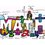# A Differentiation problem

Differentiate

$\huge x^{x^{x}}$Note by Mehul Chaturvedi
6 years, 5 months ago

This discussion board is a place to discuss our Daily Challenges and the math and science related to those challenges. Explanations are more than just a solution — they should explain the steps and thinking strategies that you used to obtain the solution. Comments should further the discussion of math and science.

When posting on Brilliant:

• Use the emojis to react to an explanation, whether you're congratulating a job well done , or just really confused .
• Ask specific questions about the challenge or the steps in somebody's explanation. Well-posed questions can add a lot to the discussion, but posting "I don't understand!" doesn't help anyone.
• Try to contribute something new to the discussion, whether it is an extension, generalization or other idea related to the challenge.
• Stay on topic — we're all here to learn more about math and science, not to hear about your favorite get-rich-quick scheme or current world events.

MarkdownAppears as
*italics* or _italics_ italics
**bold** or __bold__ bold
- bulleted- list
• bulleted
• list
1. numbered2. list
1. numbered
2. list
Note: you must add a full line of space before and after lists for them to show up correctly
paragraph 1paragraph 2

paragraph 1

paragraph 2

[example link](https://brilliant.org)example link
> This is a quote
This is a quote
    # I indented these lines
# 4 spaces, and now they show
# up as a code block.

print "hello world"
# I indented these lines
# 4 spaces, and now they show
# up as a code block.

print "hello world"
MathAppears as
Remember to wrap math in $$ ... $$ or $ ... $ to ensure proper formatting.
2 \times 3 $2 \times 3$
2^{34} $2^{34}$
a_{i-1} $a_{i-1}$
\frac{2}{3} $\frac{2}{3}$
\sqrt{2} $\sqrt{2}$
\sum_{i=1}^3 $\sum_{i=1}^3$
\sin \theta $\sin \theta$
\boxed{123} $\boxed{123}$

## Comments

Sort by:

Top Newest

$let\quad \\ \quad { x }^{ { x }^{ x } }=y\quad \quad AND\quad { x }^{ x }=z\\ now\quad let\quad us\quad find\quad the\quad value\quad of\quad \frac { dz }{ dx\\ } \\ xlogx=logz\\ \frac { d }{ dx } xlogx=\frac { d }{ dx } logz\\ applying\quad the\quad product\quad rule\\ \frac { x }{ x } \quad +logx(1)=\frac { \frac { dz }{ dx } }{ z } \\ { x }^{ x }(1+logx)=\frac { dz }{ dx } \quad .........1.\\ \\ now\quad let\quad us\quad find\quad the\quad value\quad of\quad \frac { dy }{ dx } \\ { x }^{ x }logx=logy\\ \frac { d }{ dx } { x }^{ x }logx=\frac { d }{ dx } logy\\ applying\quad he\quad product\quad rule\\ \frac { { x }^{ x } }{ x } \quad +\quad logx(\frac { d }{ dx } { x }^{ x })=\frac { 1 }{ y } (\frac { dy }{ dx } )\\ \frac { { x }^{ x } }{ x } +\quad logx({ x }^{ x })(1\quad +\quad logx)=\frac { 1 }{ y } (\frac { dy }{ dx } )\\ \\ y(\frac { { x }^{ x } }{ x } \quad +\quad logx({ x }^{ x })(1\quad +\quad logx))=\frac { dy }{ dx } \\ ({ x }^{ { x }^{ x } })({ x }^{ x })(\frac { 1 }{ x } \quad +\quad logx(1\quad +\quad logx)=\frac { dy }{ dx } \\$

- 6 years, 5 months ago

Log in to reply

How will I post my answer in this format? Please help me. I can't post my solution.

- 6 years, 5 months ago

Log in to reply

when you post something there is an option which says'FORMATTING GUIDE' click on that and than below it you'll see an option of 'EASY MATH EDITOR' click on that and than it will take to an app through which you can write like this.(use google chrome)

- 6 years, 5 months ago

Log in to reply

But u should use latex only for maths symbols

- 6 years, 5 months ago

Log in to reply

yes i've got this....but i cant post the picture or the text...

- 6 years, 5 months ago

Log in to reply

- 6 years, 5 months ago

Log in to reply

But I have a solution. So I'm posting a new note in my profile.

- 6 years, 5 months ago

Log in to reply

Take log and differentiate and for x^x again take log and differentiate

- 6 years, 5 months ago

Log in to reply

Hey buddy listen up! This sum is quite an easy one but your solution has made it far too complex. Simply take y=x^x^x and then take log on both sides then simply differentiate and voila! You will get your result in a jiffy by applying the product rule.

- 6 years, 4 months ago

Log in to reply

×

Problem Loading...

Note Loading...

Set Loading...